View Part: 1 2 3 4 5 6 7 8 9 10 11 12

PART 2 - THE GOLDEN SECTION

The Greek letter phi (φ) signifies the golden section, also known as the divine proportion of 1.618 to one. The mathematical formula for φ is the square root of five plus one, divided by two. If a line is divided with the ratio of φ between the longer segment and the shorter segment, the ratio between the whole line and the longer segment is also φ. Given a length of one for the shorter segment, the length of the longer segment is φ and the length of the entire segment is φ plus one. φ plus one is also equal to φ²:

1.618 + 1 = 2.618 and
1.618 x 1.618 = 2.618

Given a length of one for the longer segment, the shorter segment is 1/φ (.618) and the length of the entire segment is φ. 1/φ is also equal to φ minus one:

1 ÷ 1.618 = .618 and
1.618 minus 1 = .618

There is also an extremely close mathematical relationship between φ and π expressed as φ² x 6 = π x 5 (2.618 times 6, divided by 5, equals 3.1416). There is an ongoing dispute about whether or not there was an ancient awareness of φ and π. In the ancient world, φ existed naturally in the proportions and growth rates of plant and animal species, as well as spirals ranging from microscopic to flowering plants and seashells to the spiral arms of the galaxy. The π ratio between the diameter and the circumference of a circle also existed naturally in the ancient world. It has also been shown that φ and π existed in many man-made ancient buildings, including the great pyramid of Giza.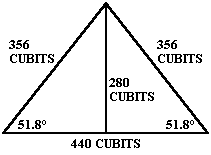The ancient Egyptian royal cubit, equal to 20.625 English inches, was used to build the great pyramid. The height of the pyramid is 280 cubits and the baselength of the sides at ground level is 440 cubits. The ratio between the height and two baselengths of the pyramid is an accurate expression of π (880/280 = 3.1428). The slant height of the pyramid is 356 cubits. The ratio between the baselength of the pyramid and the two slant heights that form the pyramid triangle is an accurate expression of φ (712/440 = 1.618).

Angkor Wat is 4,745 miles from the Great Pyramid and the Great Pyramid is 7,677 miles from Nazca. This is a precise expression of φ:

 4,745 x 1.618 = 7,677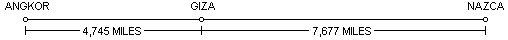Ninety miles northeast of Angkor Wat are the Angkor temples at Prassat Preah Vihear. Preah Vihear is 4754 miles from the Great Pyramid. The line of ancient sites crosses over the Great Pyramid and Angkor Vihear.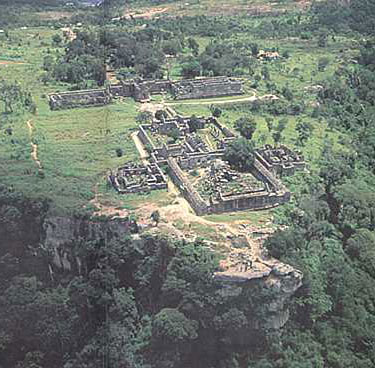Angkor Prassat Preah Vihear

Twenty five miles northwest of the city of Nazca is a figure known as the Hummingbird. The Hummingbird is 7,692 miles from the Great Pyramid. The line of ancient sites also crosses over the Hummingbird.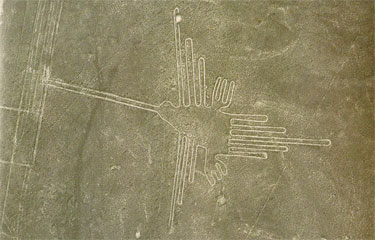The Hummingbird

The relationship between the distances from Angkor Vihear to the Great Pyramid and from the Great Pyramid to the Nazcan Hummingbird is also a precise expression of φ:

4,754 x 1.618 = 7,692

Because the Hummingbird and Angkor Vihear are antipodal sites, with a distance between them of one-half of the circumference of the earth, two Golden Section relationships between these three sites are shown by the circumference of the earth along the line of ancient sites: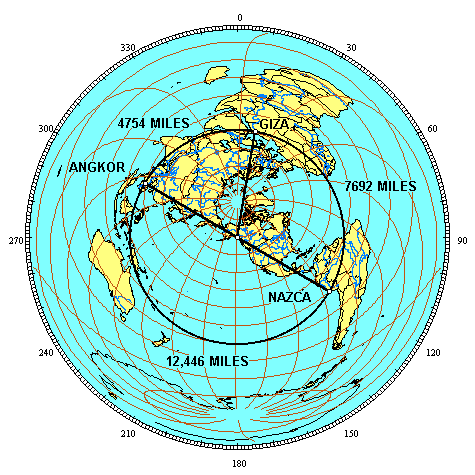4,754 x 1.618 = 7,692

4,754 + 7,692 = 12,446, and

7,692 x 1.618 = 12,446

These Golden Section relationships may also be diagramed on a straight line: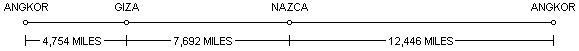The line of ancient sites is a line, from the perspective of the first illustration in Part One, and it is a circle, from the perspective of the azimuthal projection above. The line and the circle are found in the greek letter φ and the number 10. Zero and one are also the first two numbers and the only two numbers in the binary code.

The φ relationships between these sites are reflected repeatedly in the first 500 Fibonacci numbers. The first three prime numbers, 2, 3 and 5, approximate the intervals along the circumference of 20%, 30% and 50%, between these three sites. This same percentage of the circumference relationship, accurate to three digits, is found in Fibonacci numbers 137-139:

 Percentage of circumference: First three digits of Fibonacci numbers: Angkor to Giza: 19.1% #137: 191... (Prime) Giza to Nazca: 30.9% #138: 309... Nazca to Angkor: 50.0% #139: 500...

The next prime Fibonacci number after #137 is #359. The distances between these sites, in miles, is reflected by Fibocacci numbers 359-361, accurately to five digits:

 Distance between sites: First five digits of Fibonacci numbers: Angkor to Giza: 4,754 miles #359: 47542... (Prime) Giza to Nazca: 7,692 miles #360: 76924... Nazca to Angkor: 12,446 miles #361: 12446...